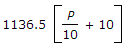# Civil Engineering - Water Supply Engineering

### Exercise :: Water Supply Engineering - Section 3

31.

The fire demand for ascertaining the empirical formulaknown as

 A. Kuichling's formula B. Buston's formula C. Freeman formula D. Under Writers formula E. None of these.

Explanation:

No answer description available for this question. Let us discuss.

32.

To detect the turbidity of the order of 0 to 1000 P.P.M. the instrument used is

 A. turbidimeter B. Jackson turbidimeter C. Baylis turbidimeter D. Hallige turbidimeter.

Explanation:

No answer description available for this question. Let us discuss.

33.

Standard process of chlorination of water, is done by

 A. plain chlorination B. pre-chlorination C. post-chlorination D. double chlorination.

Explanation:

No answer description available for this question. Let us discuss.

34.

If the chosen diameter of a pipe, is less than the economical diameter

 A. cost of pipe will be less B. head loss will be high C. cost of pumping will be more than saving D. all the above.

Explanation:

No answer description available for this question. Let us discuss.

35.

Water gets evaporated from water surfaces and land surfaces, get converted into water drops at lower temperatures, flows over ground surface and finally meets its source, i.e. lake, sea, etc. This entire process is generally known as

 A. hydrological cycle B. water cycle C. evaporation and precipitation cycle D. all the above.